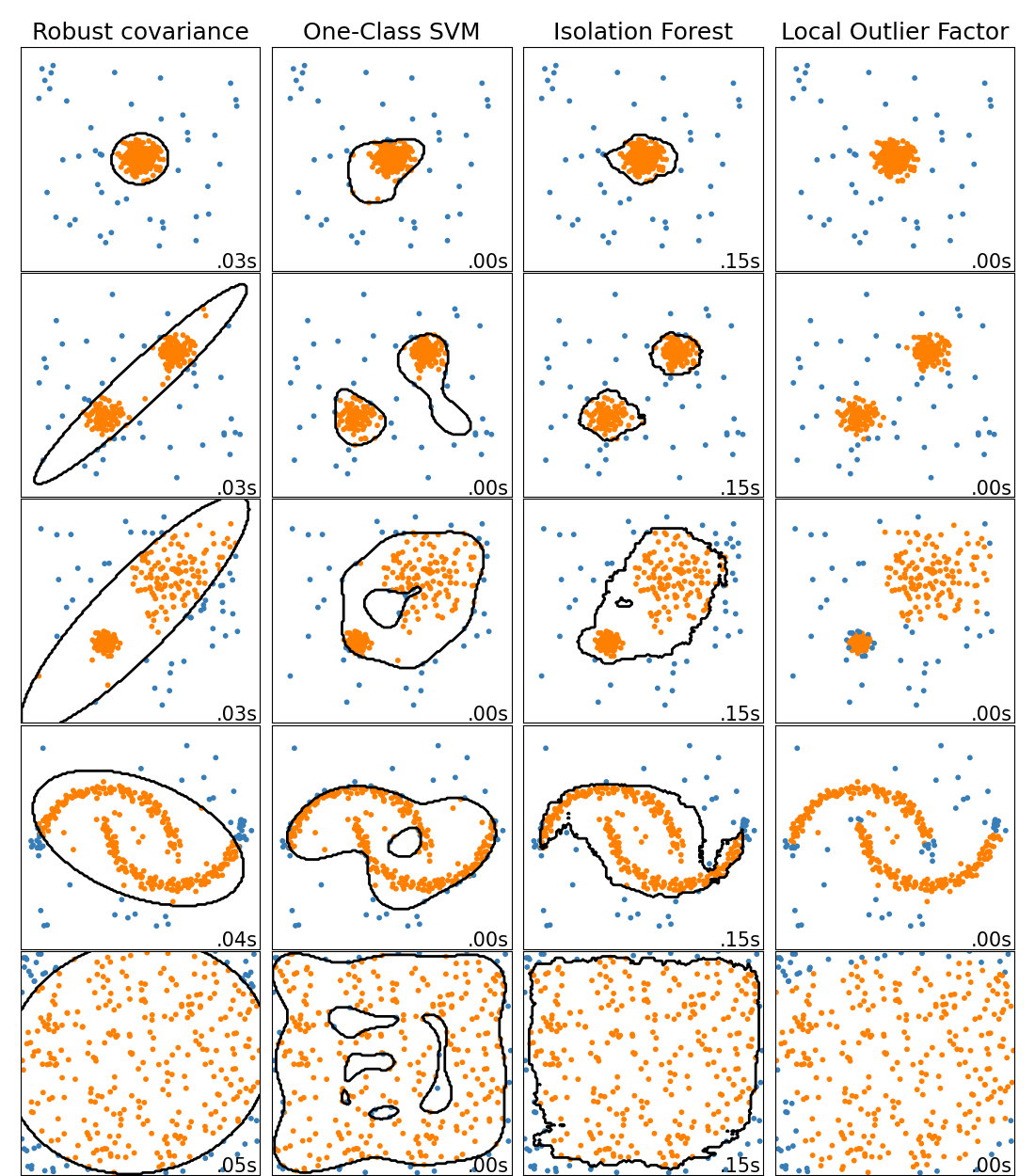# How to create a Matplotlib Pie chart annotated with percentages and labels?

In this short post, we look at how to create a pie chart as follows:

The key code blocks are as follows:

The function to compute the percentages:

`def my_autopct(pct):    return ('%.2f' % pct)`

The function to assign labels:

`def get_new_labels(sizes, labels):    new_labels = [label for size, label in zip(sizes, labels)]    return new_labels`

How do you show the labels only for the significant shares (especially if we have many entries in the bar chart)?

--

--

# How to add counts to Python Matplotlib Pandas Dataframe Bar Charts

This short post demonstrates how you can add the count values to the top of the bars in a bar chart.

Here’s the code snippet:

The high-level idea is as follows:

1. Create the chart and save to ax
2. Use the following code snippet to annotate with the counts:
`for p in ax.patches:    ax.annotate(str(p.get_height()), (p.get_x() * 1.005, p.get_height() * 1.005))`

--

--

# What’s the difference between novelty detection and outlier detection?

In novelty detection, you have a dataset that contains only good data and you are trying to check if new observations are similar to the the good data. In other words, our goal is to check if new observations are outliers.

In outlier detection, you dataset may already have outliers and your goal is to identify such outliers.

Both novelty detection and outlier detection are used to detect anomalies.

Outlier detection is an unsupervised anomaly detection algorithm.

Novelty detection is a semi-supervised anomaly detection algorithm.Outlier detection methods available in scikit-learn (LOF does not have a decision boundary as it does not have a predict method when used as an outlier detection algorithm)

--

--

# When would K-means clustering fail to give good results?

• Data points contain outliers
• Data points in non-convex/non-round shapes
• Data points with different densities

--

--

# What would be the best number of clusters for the following dendrogram?

You select the y that has the maximum range that does not change the cluster assignment.

--

--

# Given a dataset, what could change the shape of a dendrogram?

• Proximity function used
• Number of features used
• Number of data points used (sampling)

--

--

# How can Clustering (Unsupervised Learning) be used to improve the accuracy of Linear Regression model (Supervised Learning)?

• A clustering algorithm groups data points into n distinct groups. If these clusters are far apart and there are a lot of data, it may be a good idea to try creating a different regression models for different groups.
• Cluster information could be used as variables in the regression model as well. For example, cluster centroid value, cluster id, and cluster size could be utilized as three additional variables to the model.

--

--